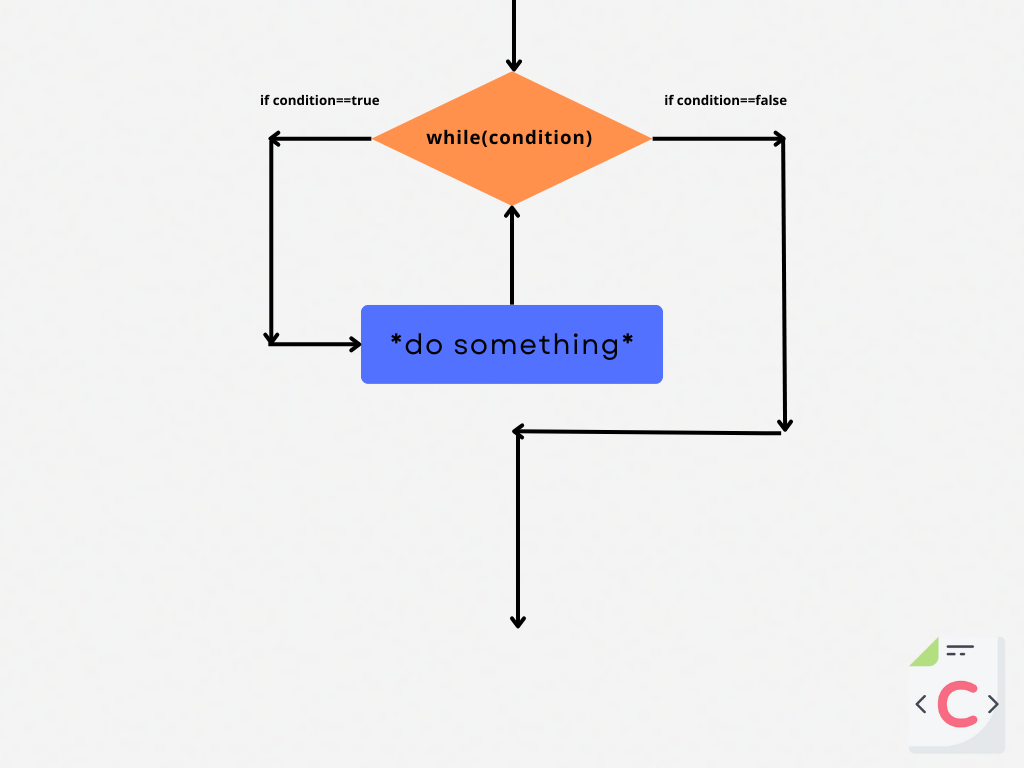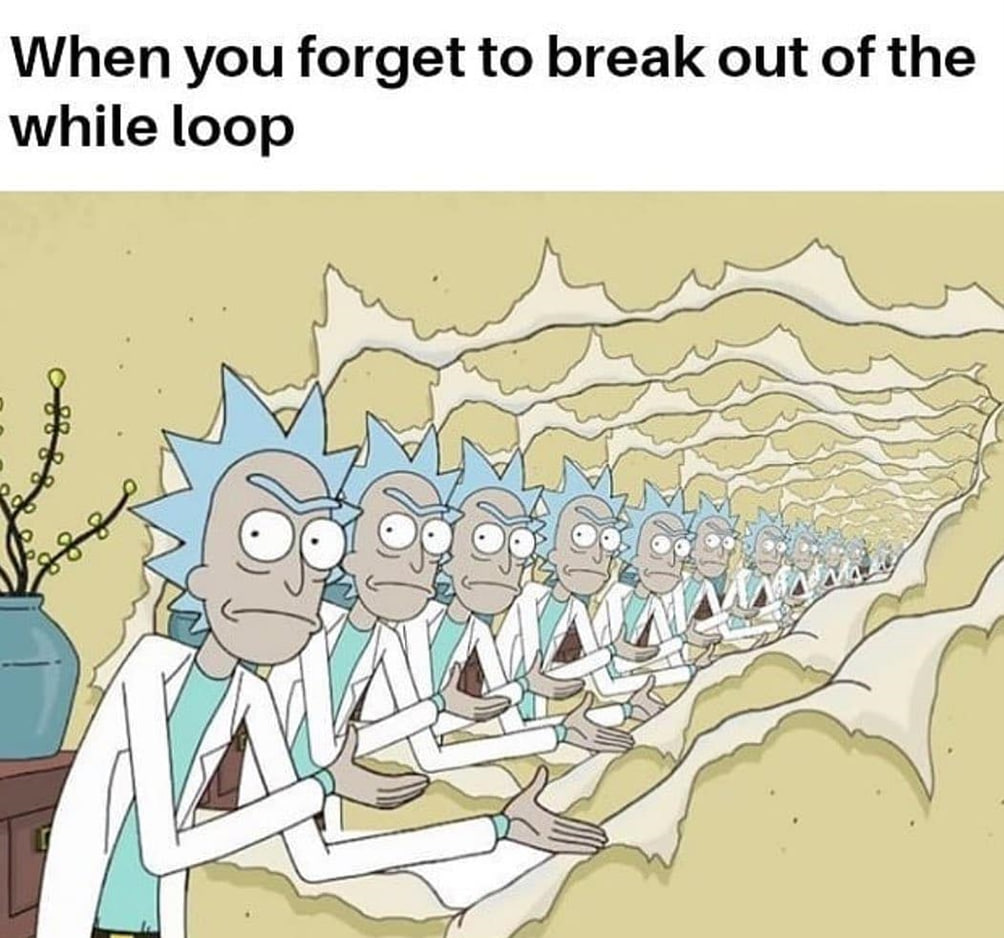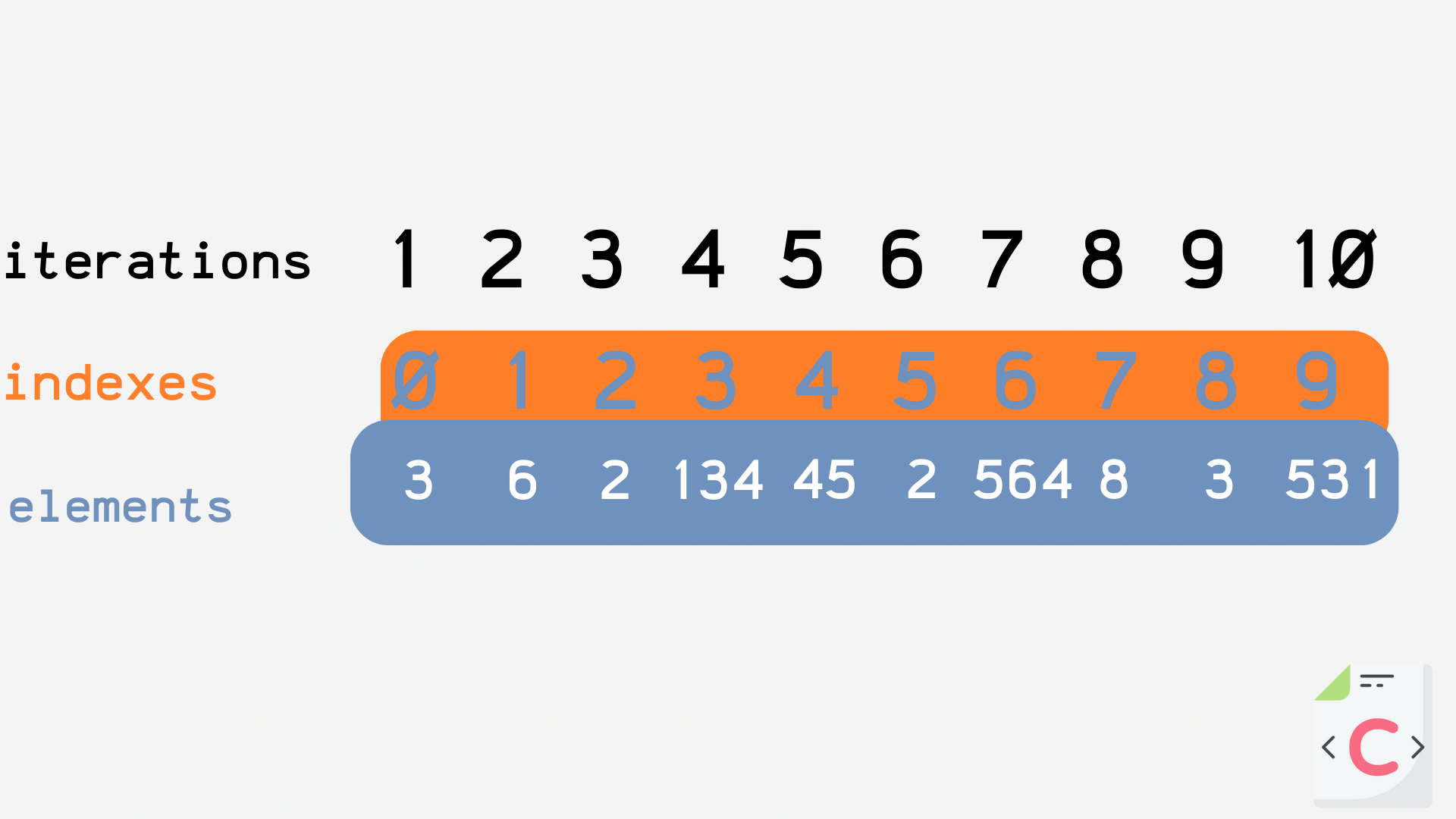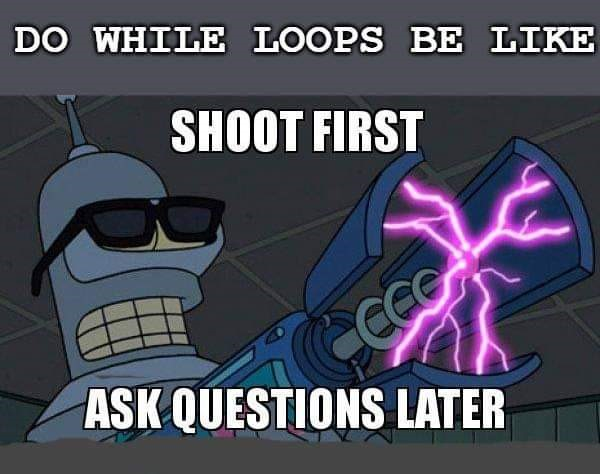Course Content

C Basics

##While, Do...While

Let's imagine a situation where we need to loop some processes, for example, reading information from a sensor, searching for passwords, or counting words in a line. For such situations, loops are used. With loops, you can execute blocks of code tens, hundreds, thousands, and hundreds of thousands of times. Loops are one of the fundamental concepts in programming, so it's critical to understand. This course will cover the basic `while` loop, `do...while` loop, `for` loop.

### While Loop

This loop will run as long as some condition is met, and as soon as the condition is no longer met, the loop will end.An elementary example of using a loop is to print the number of iterations:Main.cIn order to stop this loop, you need to create a condition to stop it. The simplest condition is the counter (the number of loop iterations).

Note

An iteration is one pass through a loop. That is, if the loop executed the code block 10 times, it means there were 10 iterations.

The `iterations++;` line is key because it is the one that increments the counter (`int iterations`) with each iteration, and the counter creates conditions for the end of the loop.

Note

Always create conditions for exiting the loop, otherwise, the loop will be infinite, and your position as a developer will be finite.Let's write a program to display the elements of an integer array:Main.cLet's pay attention to the expression `array[i]`. In this case, the `i` variable indicates the index of the elements of the `array[]` array.

Note

An iteration is the number of iterations of the loop.

With each iteration, the variable `i` is incremented by 1, which means that with each iteration, the expression `array[i]` will access the next element of the array:### Do...While

The `do...while` loop differs from the `while` loop only in that the `do...while` loop is guaranteed to execute at least once, even if the condition is false.Example:Main.cSuch a loop can be used to create a simple user interface, such as asking for a password. If the password is incorrect, the program will ask for it again until the user enters the correct password:Main.c